Example Questions

1 2 151 152 153 154 155 156 157 159 Next →

Example Question #1 : How To Solve For A Variable As Part Of A Fraction

If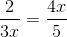, and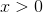what is the value of?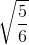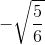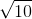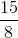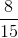Explanation:

Start by cross multiplying which gives us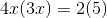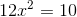.

Divide both sides by 12 and simplify which gives us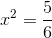.

Since, we only take the positive square root as the solution,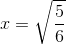.

Example Question #1152 : Algebra

If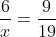, then what is the value of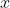?

7/12

9/114

38/3

3/38

none of these

38/3

Explanation:

cross multiply:

(6)(19) = 9x

114=9x

x = 38/3

1 2 151 152 153 154 155 156 157 159 Next →

Tired of practice problems?

Try live online GRE prep today.